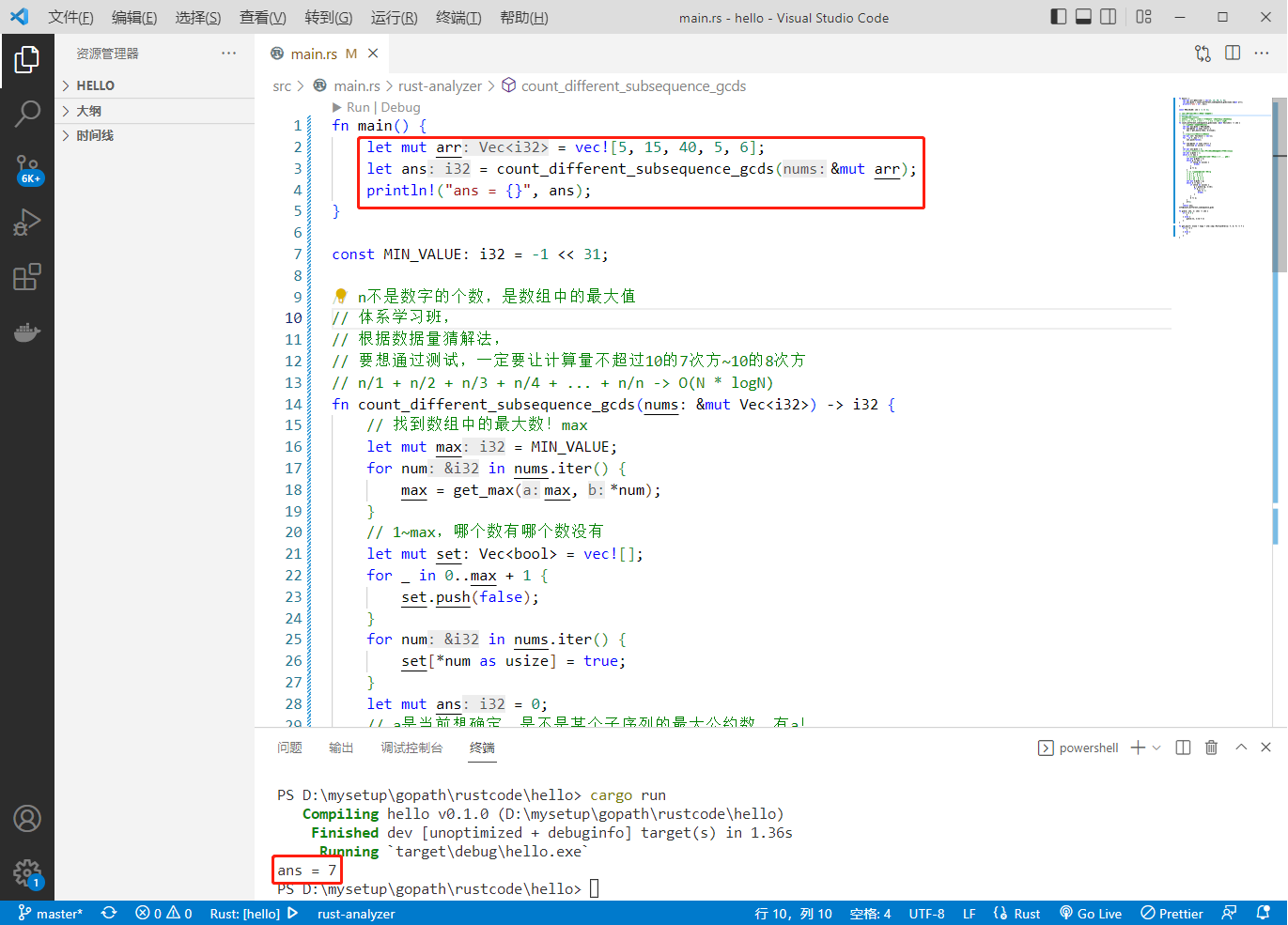# 2022-09-07：给你一个由正整数组成的数组 nums 。 数字序列的 最大公约数 定义为序列中所有整数的共

2022-09-07：给你一个由正整数组成的数组 nums 。

n/1 + n/2 + n/3 + n/4 + … + n/n 收敛于 O(N * logN)，N是最大值，当做结论记住。

``````fn main() {
let mut arr = vec![5, 15, 40, 5, 6];
let ans = count_different_subsequence_gcds(&mut arr);
println!("ans = {}", ans);
}

const MIN_VALUE: i32 = -1 << 31;

// n不是数字的个数，是数组中的最大值
// 体系学习班，
// 根据数据量猜解法，
// 要想通过测试，一定要让计算量不超过10的7次方~10的8次方
// n/1 + n/2 + n/3 + n/4 + ... + n/n -> O(N * logN)
fn count_different_subsequence_gcds(nums: &mut Vec<i32>) -> i32 {
// 找到数组中的最大数！max
let mut max = MIN_VALUE;
for num in nums.iter() {
max = get_max(max, *num);
}
// 1~max，哪个数有哪个数没有
let mut set: Vec<bool> = vec![];
for _ in 0..max + 1 {
set.push(false);
}
for num in nums.iter() {
set[*num as usize] = true;
}
let mut ans = 0;
// a是当前想确定，是不是某个子序列的最大公约数，有a！
let mut a = 1;
while a <= max {
// 1)找到，离a最近的，a的倍数！1 2 3 ... g就是
let mut g = a;
while g <= max {
if set[g as usize] {
break;
}
g += a;
}
// 2) 找到了a最近的倍数，g
// g + 0 , g ?= a
// g + a , g ?= a
// g + 2a , g ?= a
// g + 3a , g ?= a
let mut b = g;
while b <= max {
if set[b as usize] {
g = gcd(g, b);
if g == a {
ans += 1;
break;
}
}
b += a;
}
a+=1;
}
return ans;
}

fn gcd(m: i32, n: i32) -> i32 {
if n == 0 {
m
} else {
gcd(n, m % n)
}
}

fn get_max<T: Clone + Copy + std::cmp::PartialOrd>(a: T, b: T) -> T {
if a > b {
a
} else {
b
}
}
``````(=￣ω￣=)··· 暂无内容！

106

5

6

3